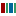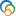The Library of Congress > Linked Data Service > LC Subject Headings (LCSH)

# Diophantine equations

• ### Variants

• Diophantic equations
• Equations, Diophantic
• Equations, Diophantine
• Equations, Indefinite
• Equations, Indeterminate
• Indefinite equations
• Indeterminate equations

• ### Closely Matching Concepts from Other Schemes

•diophantine equationLabel from public data source Wikidata
•Diophantine equations• ### Sources

• found: Work cat.: Schmidt, W.M. Diophantine approximations and diophantine equations, c1991.
• found: Eisenreich. Mathematik(Diophantine equation; Diophantic (indeterminate) equations; NT)
• found: Encyc. dict. math.
• found: Math. subj. classif.(Number theory, 11-XX; Diophantine equations, 11Dxx)
• found: CompuMath cit. index.
• found: Encyc. math.(Diophantine equations; indefinite equations)

• QA242
• ### Change Notes

• 1992-02-28: new
• 2012-03-30: revised

# Suggest terminology

The LC Linked Data Service welcomes any suggestions you might have about terminology used for a given heading or concept.

Would you like to suggest a change to this heading?

Please provide your name, email, and your suggestion so that we can begin assessing any terminology changes.

Fields denoted with an asterisk (*) are required.